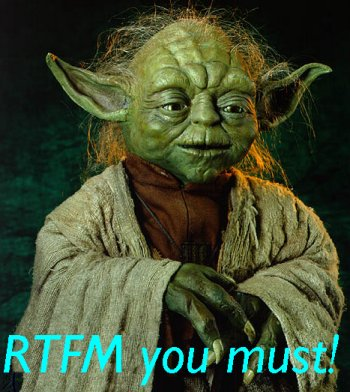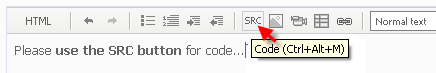874
forexparvenu

as i mentioned above, i wish to make ea with stochastic coding.

the problem is how to add indicator code into ea ??17239

albert_lim83:

the problem is how to add indicator code into ea ??

You can, with certain limitations, but using iCustom is a whole lot easier.

But if you really want to: Transferring an Indicator Code into an Expert Advisor Code. Indicator Structure - MQL4 Articles6659

For Stochastic it is not needed iCustom

 double iStochastic( string symbol, int timeframe, int %Kperiod, int %Dperiod, int slowing, int method, int price_field, int mode, int shift)
Calculates the Stochastic oscillator and returns its value.
Parameters:
 symbol - Symbol the data of which should be used to calculate indicator. NULL means the current symbol. timeframe - Timeframe. It can be any of Timeframe enumeration values. 0 means the current chart timeframe. %Kperiod - %K line period. %Dperiod - %D line period. slowing - Slowing value. method - MA method. It can be any ofMoving Average method enumeration value. price_field - Price field parameter. Can be one of this values: 0 - Low/High or 1 - Close/Close. mode - Indicator line index. It can be any of the Indicators line identifiers enumeration value. shift - Index of the value taken from the indicator buffer (shift relative to the current bar the given amount of periods ago).
Sample:
```  if(iStochastic(NULL,0,5,3,3,MODE_SMA,0,MODE_MAIN,0)>iStochastic(NULL,0,5,3,3,MODE_SMA,0,MODE_SIGNAL,0))
return(0);
```
A lot of coding help is here to find Alphabetic Index of MQL4 Functions https://www.mql5.com/en/forum/122679874
forexparvenu

also using the same code as the way adding stochastic ?172396659

albert_lim83:

also using the same code as the way adding stochastic ?

iCustom() or iRVI()20759

albert_lim83:

also using the same code as the way adding stochastic ?

1.2. Detailed explanation of iCustom - MQL4 forum874
forexparvenu

i trying to add RVI indicator into escape EA, but failure...

it show

expression on global scope not allowed

function definition unexpected

unbalanced parentheses

how to fix these error ??874
forexparvenu

here the code i did this morning.

anyone can help me to fix it ??

//+------------------------------------------------------------------+
int init()
{
//---- indicator buffers mapping
SetIndexBuffer(0,Buffer);
SetIndexBuffer(1,SignalBuffer);
//---- indicator line
SetIndexStyle(0,DRAW_LINE);
SetIndexStyle(1,DRAW_LINE);
//---- drawing settings
SetIndexDrawBegin(0,RVIPeriod+3);
SetIndexDrawBegin(1,RVIPeriod+7);
//---- name for DataWindow and indicator subwindow label
IndicatorShortName("RVI("+RVIPeriod+")");
SetIndexLabel(0,"RVI");
SetIndexLabel(1,"RVIS");
//---- initialization done
return(0);
}
{
double bid =MarketInfo(Symbol(),MODE_BID); // Request for the value of Bid
double point =MarketInfo(Symbol(),MODE_POINT);//Request for Point
return; // Exit start()
if(AccountFreeMargin()<100)
Print("We have no money. Free Margin = ", AccountFreeMargin());
return(0);
}

int start()
{
{
int i,j,nLimit,nCountedBars;
double dValueUp,dValueDown,dNum,dDeNum;
//----
if(Bars<=RVIPeriod+8) return(0);
//----
nCountedBars=IndicatorCounted();
//---- check for possible errors
if(nCountedBars<0) return(-1);
//---- last counted bar will be recounted
nLimit=Bars-RVIPeriod-4;
if(nCountedBars>RVIPeriod+4)
nLimit=Bars-nCountedBars;
//---- RVI counted in the 1-st buffer
for(i=0; i<=nLimit; i++)
{
dNum=0.0;
dDeNum=0.0;
for(j=i; j<i+RVIPeriod; j++)

{
dValueUp=((Close[j]-Open[j])+2*(Close[j+1]-Open[j+1])+2*(Close[j+2]-Open[j+2])+(Close[j+3]-Open[j+3]))/6;
dValueDown=((High[j]-Low[j])+2*(High[j+1]-Low[j+1])+2*(High[j+2]-Low[j+2])+(High[j+3]-Low[j+3]))/6;
dNum+=dValueUp;
dDeNum+=dValueDown;
}
if(dDeNum!=0.0)
Buffer[i]=dNum/dDeNum;
else
Buffer[i]=dNum;
}
//---- signal line counted in the 2-nd buffer
nLimit=Bars-RVIPeriod-7;
if(nCountedBars>RVIPeriod+8)
nLimit=Bars-nCountedBars+1;
for(i=0; i<=nLimit; i++)
SignalBuffer[i]=(Buffer[i]+2*Buffer[i+1]+2*Buffer[i+2]+Buffer[i+3])/6;
//----
return(0);
}
{
if (Close<i)
return(0);

OrderSend(Symbol,LotSize,Bid,StopLoss,TakeProfit,0,0);
if (Close>i)
OpenSell();
return(0);
}

double GetSizeLot() { return(LotSize); }
double GetTakeProfitSell() { return(Bid-TakeProfit*Point); }
string GetCommentForOrder() { return();}

return(0); }17239

Before posting please read some of the other threads . . . then you would have seen numerous requests like this one:

Please use this to post code . . . it makes it easier to read.17239

albert_lim83:

here the code i did this morning.

anyone can help me to fix it ??

You can't use Indicator code in an EA . . . read the thread I posted a link to. You have to have equal numbers of { and } also equal numbers of ( and ) . . . . haven't I already said this once ? https://www.mql5.com/en/forum/139447 I guess you don't bother to learn from other posts ?